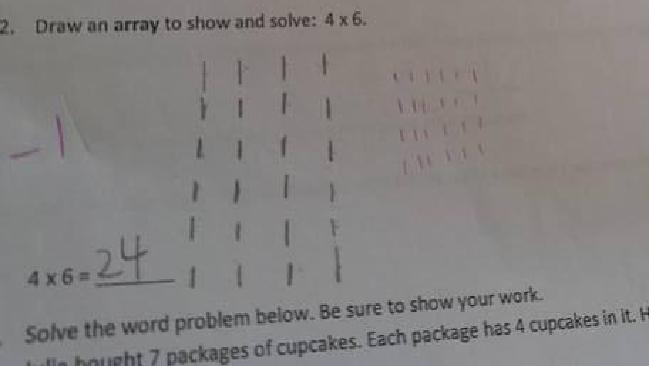A third grade maths quiz has caused outrage online after it showed a student was marked down even though they gave the correct answer.

The test question, posted to Reddit, was marked down for the way the student calculated the right answer.

The student answered 5+5+5=15 when asked to calculate 5 x 3 however the accurate advised answer by the teacher was 3+3+3+3+3=15.

Question two was also ‘wrong’.The student was asked to draw an array to solve 4×6.

For the answer, the student drew six rows of four dashes however the teacher suggested the correct answer was 4 rows of six dashes.

Advertisement
Advertisement

New York High School math and physics teacher Frank Noschese said the questions were part of the Common Core standards – an educational initiative in the US.

“The standards just lay out what kids should know and be able to do, not actual lessons” he told Tech Insider

Mr Noschese said while Common Core stipulates goals for knowledge in each grade, the specific interpretation of these standards is up to the individual state, districts and teachers.

“If the teacher specifically said ‘5×3 means five groups of three and 4×6 means four groups of six’ these answers are wrong because of the teacher’s forced interpretation … But mathematically, what the kid did is also valid. Kids likely know that five groups of three is equal to three groups of five.”

Source: News.com.au

Advertisement
Advertisement
Love this? You’ll love this bit from Will & Woody – catch the boys weekdays from 4PM on KIIS 1065